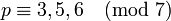# Linear representation theory of alternating group:A7

## Contents

View linear representation theory of particular groups | View other specific information about alternating group:A7

## Summary

Item Value
degrees of irreducible representations over a splitting field 1,6,10,10,14,14,15,21,35
grouped form: 1 (1 time), 6 (1 time), 10 (2 times), 14 (2 times), 15 (1 time), 21 (1 time), 35 (1 time)
maximum: 35, lcm: 210, number: 9, sum of squares: 2520
Minimal splitting field, i.e., field of realization of all irreducible representations (characteristic zero)$\mathbb{Q}(\omega + \omega^2 + \omega^4)$ where$\omega$ is a primitive seventh root of unity. This is the same as$\mathbb{Q}(\sqrt{-7})$
Quadratic extension of$\mathbb{Q}$
Same as field generated by character values
Condition for a field of characteristic not 2,3,5, or 7, to be a splitting field -7 should be a square in the field.
Minimal splitting field, i.e., field of realization of all irreducible representations in prime characteristic$p \ne 2,3,5,7$ Case$p \equiv 1,2,4 \pmod 7$: prime field$\mathbb{F}_p$
Case$p \equiv 3,5,6 \pmod 7$: quadratic extension$\mathbb{F}_{p^2}$
Smallest size splitting field field:F11

## Family contexts

Family name Parameter values General discussion of linear representation theory of family
alternating group$A_n$ degree$n = 7$, i.e., the group$A_7$ linear representation theory of alternating groups

## GAP implementation

### Degrees of irreducible representations

The degrees of irreducible representations can be computed using GAP's CharacterDegrees, CharacterTable, and AlternatingGroup functions:

gap> CharacterDegrees(AlternatingGroup(7));
[ [ 1, 1 ], [ 6, 1 ], [ 10, 2 ], [ 14, 2 ], [ 15, 1 ], [ 21, 1 ], [ 35, 1 ] ]

This means there is 1 degree 1 irreducible, 1 degree 6 irreducible, 2 degree 10 irreducibles, 2 degree 14 irreducibles, and 1 irreducible each of degrees 15, 21, 35.

### Character table

The characters of irreducible representations can be computed using the Irr, CharacterTable, and CharacterDegree functions:

gap> Irr(CharacterTable(AlternatingGroup(7)));
[ Character( CharacterTable( Alt( [ 1 .. 7 ] ) ), [ 1, 1, 1, 1, 1, 1, 1, 1, 1 ] ), Character( CharacterTable( Alt( [ 1 .. 7 ] ) ),
[ 6, 2, 3, -1, 0, 0, 1, -1, -1 ] ), Character( CharacterTable( Alt( [ 1 .. 7 ] ) ), [ 10, -2, 1, 1, 1, 0, 0, E(7)^3+E(7)^5+E(7)^6, E(7)+E(7)^2+E(7)^4
] ), Character( CharacterTable( Alt( [ 1 .. 7 ] ) ), [ 10, -2, 1, 1, 1, 0, 0, E(7)+E(7)^2+E(7)^4, E(7)^3+E(7)^5+E(7)^6 ] ),
Character( CharacterTable( Alt( [ 1 .. 7 ] ) ), [ 14, 2, 2, 2, -1, 0, -1, 0, 0 ] ), Character( CharacterTable( Alt( [ 1 .. 7 ] ) ),
[ 14, 2, -1, -1, 2, 0, -1, 0, 0 ] ), Character( CharacterTable( Alt( [ 1 .. 7 ] ) ), [ 15, -1, 3, -1, 0, -1, 0, 1, 1 ] ),
Character( CharacterTable( Alt( [ 1 .. 7 ] ) ), [ 21, 1, -3, 1, 0, -1, 1, 0, 0 ] ), Character( CharacterTable( Alt( [ 1 .. 7 ] ) ),
[ 35, -1, -1, -1, -1, 1, 0, 0, 0 ] ) ]